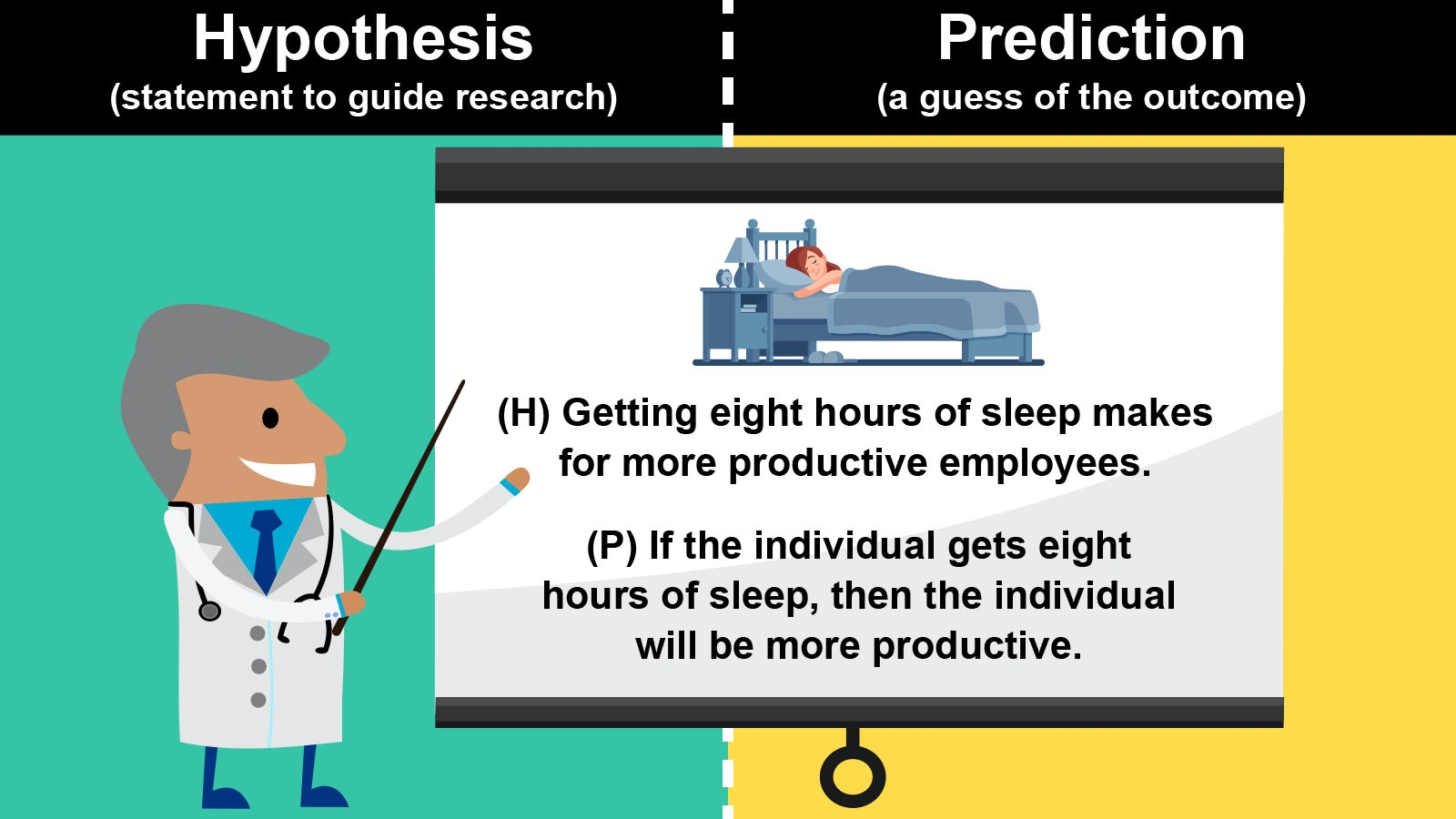# Difference Between Making a Hypothesis and Prediction

Hypothesis and prediction are commonly used interchangeably. But are they the same? Learning the difference between hypothesis and prediction comes down to science. Explore hypothesis vs. prediction through examples of each one.difference between hypothesis and prediction

## Difference Between a Hypothesis and Prediction

Hypothesis and prediction are both a type of guess. That’s why many people get the two confused. However, the hypothesis is an educated, testable guess in science. A prediction uses observable phenomena to make a future projection. However, prophets can also make predictions based on nothing at all. To get a good understanding of how a hypothesis differs from a prediction, it’s best to look at each term individually.

## What Is a Hypothesis?

Scientists make hypotheses before doing experiments. These help guide their research for an unexplained phenomenon. Therefore, a hypothesis is an explanation for why a specific occurrence or problem is happening. Scientists use the scientific method when creating and testing a hypothesis through experimentation.

While hypotheses come in different forms from simple to statistical, it always defines the independent and dependent variables to be tested. It also uses precise language that is testable during experiments. You could call a hypothesis a testable guess.

### Hypothesis Examples

Understanding a hypothesis can be hard. Check out some different hypothesis examples to better understand this intelligent estimation type.

• Consuming greasy high-fat content foods causes more skin oils and breakouts.

• Getting eight hours of sleep makes for more productive employees.

• Instituting relaxation sessions within the workday makes for happier employees.

• Fewer than 8 hours of sleep causes less productivity.

• Employees that are happier in their positions work harder.

All these different hypotheses clarify the variables and are testable.

## What Is a Prediction in Science?

Just like a hypothesis, a prediction is a type of guess. However, a prediction is an estimation made from observations. For example, you observe that every time the wind blows, flower petals fall from the tree. Therefore, you could predict that if the wind blows, petals will fall from the tree. Based on your observations of the wind and the tree, this is a good prediction of future behavior. Therefore, by definition, a prediction is making a statement of what will happen in the future.

In science, a prediction is what you expect to happen if your hypothesis is true. So, based on the hypothesis you’ve created, you can predict the outcome of the experiment. For example, if you hypothesize that greasy food leads to skin outbreaks, then you can write a prediction as an if, then statements like “if the person eats greasy food, then the person will have a skin outbreak.” And that’s how prediction works.

### Prediction Examples

Need a few more examples of predictions? Explore these unique predictions to clarify the difference between hypothesis and prediction.

• If the individual consumes greasy foods, then the person will have more skin oils and breakouts.

• If the individual gets eight hours of sleep, then the individual will be more productive.

• If the employer institutes a relaxation session in the workday, then the employees will be happier.

• If the individual gets fewer than 8 hours of sleep, then the individual will be less productive.

• If the employees are happier, then the workplace will be more productive.

## Hypothesis vs. Prediction

Now that you’ve seen a hypothesis and prediction in action, it’s time to break the two down in a simple table.

 Term Hypothesis Prediction Definition Explanation of a phenomenon Event that will occur if phenomenon is true What it does Explains why something happens Forecasts future event How it’s written Statement with variables If, then statement Example Cholesterol of higher than 400 leads to heart disease If someone has cholesterol of higher than 400, then they have heart disease.

## Using Predictions and Hypothesis in Science

Predictions and hypotheses work in science to help clarify an experiment. Not only are you using the hypothesis to determine the independent and dependent variables to be tested, but you are predicting what will happen if you are right.

Don’t end your learning with prediction vs. hypothesis, keep this scientific win going by looking at how to create a hypothesis